Courses

# NCERT Solutions - Atoms Class 12 Notes | EduRev

## Class 12 : NCERT Solutions - Atoms Class 12 Notes | EduRev

The document NCERT Solutions - Atoms Class 12 Notes | EduRev is a part of the Class 12 Course Physics For JEE.
All you need of Class 12 at this link: Class 12

NCERT QUESTIONS:

(Atoms)
Ques 12.1:
Choose the correct alternative from the clues given at the end of the each statement:
(a) The size of the atom in Thomson’s model is .......... the atomic size in Rutherford’s model. (much greater than/no different from/much less than.
(b) In the ground state of .......... electrons are in stable equilibrium, while in .......... electrons always experience a net force.
(Thomson’s model/ Rutherford’s model.)
(c) A classical atom based on .......... is doomed to collapse.
(Thomson’s model/ Rutherford’s model.)
(d) An atom has a nearly continuous mass distribution in a .......... but has a highly non-uniform mass distribution in ..........
(Thomson’s model/ Rutherford’s model.)
(e) The positively charged part of the atom possesses most of the mass in .......... (Rutherford’s model/both the models.)
Ans: (a) The sizes of the atoms taken in Thomson’s model and Rutherford’s model have the same order of magnitude.
(b) In the ground state of Thomson’s model, the electrons are in stable equilibrium. However, in Rutherford’s model, the electrons always experience a net force.
(c) A classical atom based on Rutherford’s model is doomed to collapse.
(d) An atom has a nearly continuous mass distribution in Thomson’s model, but has a highly non-uniform mass distribution in Rutherford’s model.
(e) The positively charged part of the atom possesses most of the mass in both the models.

Ques 12.2:
Suppose you are given a chance to repeat the alpha-particle scattering experiment using a thin sheet of solid hydrogen in place of the gold foil. (Hydrogen is a solid at temperatures below 14 K.) What results do you expect?
Ans: In the alpha-particle scattering experiment, if a thin sheet of solid hydrogen is used in place of a gold foil, then the scattering angle would not be large enough. This is because the mass of hydrogen (1.67 × 10−27 kg) is less than the mass of incident α−particles (6.64 × 10−27 kg). Thus, the mass of the scattering particle is more than the target nucleus (hydrogen). As a result, the α−particles would not bounce back if solid hydrogen is used in the α-particle scattering experiment.

Ques 12.3:
What is the shortest wavelength present in the Paschen series of spectral lines?
Ans: Rydberg’s formula is given as: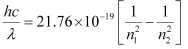Where,
h = Planck’s constant = 6.6 × 10−34 Js
c = Speed of light = 3 × 108 m/s
(n1 and n2 are integers)
The shortest wavelength present in the Paschen series of the spectral lines is given for values n1 = 3 and n2 = ∞.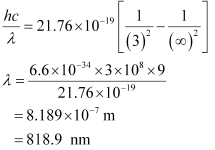Ques 12.4:
A difference of 2.3 eV separates two energy levels in an atom. What is the frequency of radiation emitted when the atom makes a transition from the upper level to the lower level?
Ans: Separation of two energy levels in an atom,
E = 2.3 eV
= 2.3 × 1.6 × 10−19
= 3.68 × 10−19 J
Let ν be the frequency of radiation emitted when the atom transits from the upper level to the lower level.
We have the relation for energy as:
E = hv
Where,
h = Planck’s constant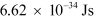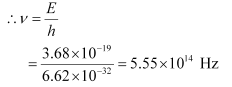Hence, the frequency of the radiation is 5.6 × 1014 Hz.

Ques 12.5:
The ground state energy of hydrogen atom is −13.6 eV. What are the kinetic and potential energies of the electron in this state?
Ans: Ground state energy of hydrogen atom, E = − 13.6 eV
This is the total energy of a hydrogen atom. Kinetic energy is equal to the negative of the total energy.
Kinetic energy = − E = − (− 13.6) = 13.6 eV
Potential energy is equal to the negative of two times of kinetic energy.
Potential energy = − 2 × (13.6) = − 27 .2 eV

Ques 12.6:
A hydrogen atom initially in the ground level absorbs a photon, which excites it to the n = 4 level. Determine the wavelength and frequency of the photon.
Ans: For ground level, n1 = 1
Let E1 be the energy of this level. It is known that E1 is related with n1 as: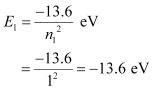The atom is excited to a higher level, n2 = 4.
Let E2 be the energy of this level.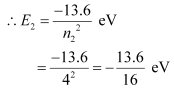The amount of energy absorbed by the photon is given as:
E = E2E1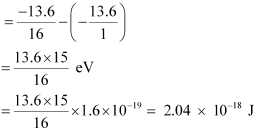For a photon of wavelengthλ, the expression of energy is written as: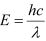Where,
h = Planck’s constant = 6.6 × 10−34 Js
c = Speed of light = 3 × 108 m/s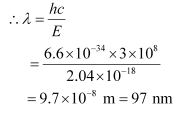And, frequency of a photon is given by the relation,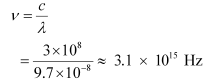Hence, the wavelength of the photon is 97 nm while the frequency is 3.1 × 1015 Hz.

Ques 12.7:
(a) Using the Bohr’s model calculate the speed of the electron in a hydrogen atom in the n = 1, 2, and 3 levels. (b) Calculate the orbital period in each of these levels.
Ans: (a) Let ν1 be the orbital speed of the electron in a hydrogen atom in the ground state level, n1 = 1. For charge (e) of an electron, ν1 is given by the relation,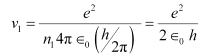Where,
e = 1.6 × 10−19 C
0 = Permittivity of free space = 8.85 × 10−12 N−1 C2 m−2
h = Planck’s constant = 6.62 × 10−34 Js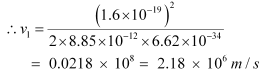For level n2 = 2, we can write the relation for the corresponding orbital speed as: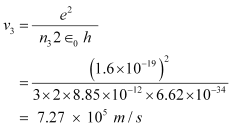And, for n3 = 3, we can write the relation for the corresponding orbital speed as: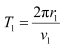Hence, the speed of the electron in a hydrogen atom in n = 1, n=2, and n=3 is 2.18 × 106 m/s, 1.09 × 106 m/s, 7.27 × 105 m/s respectively.
(b) Let T1 be the orbital period of the electron when it is in level n1 = 1.
Orbital period is related to orbital speed as: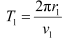Where,
r1 = Radius of the orbit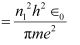h = Planck’s constant = 6.62 × 10−34 Js
e = Charge on an electron = 1.6 × 10−19 C
0 = Permittivity of free space = 8.85 × 10−12 N−1 C2 m−2
m = Mass of an electron = 9.1 × 10−31 kg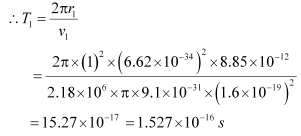For level n2 = 2, we can write the period as: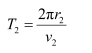Where,
r2 = Radius of the electron in n2 = 2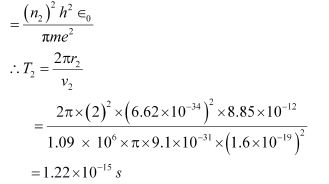And, for level n3 = 3, we can write the period as: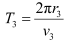Where,
r3 = Radius of the electron in n3 = 3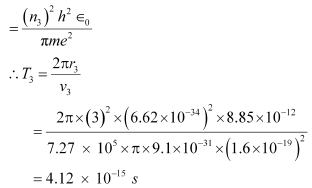Hence, the orbital period in each of these levels is 1.52 × 10−16 s, 1.22 × 10−15 s, and 4.12 × 10−15 s respectively.

Ques 12.8:
The radius of the innermost electron orbit of a hydrogen atom is 5.3 ×10−11 m. What are the radii of the n = 2 and n =3 orbits?
Ans: The radius of the innermost orbit of a hydrogen atom, r1 = 5.3 × 10−11 m.
Let r2 be the radius of the orbit at n = 2. It is related to the radius of the innermost orbit as: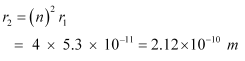For n = 3, we can write the corresponding electron radius as: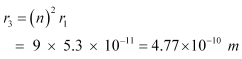Hence, the radii of an electron for n = 2 and n = 3 orbits are 2.12 × 10−10 m and 4.77 × 10−10 m respectively.

Ques 12.9:
A 12.5 eV electron beam is used to bombard gaseous hydrogen at room temperature. What series of wavelengths will be emitted?
Ans: It is given that the energy of the electron beam used to bombard gaseous hydrogen at room temperature is 12.5 eV. Also, the energy of the gaseous hydrogen in its ground state at room temperature is −13.6 eV.

When gaseous hydrogen is bombarded with an electron beam, the energy of the gaseous hydrogen becomes −13.6 12.5 eV i.e., −1.1 eV.
Orbital energy is related to orbit level (n) as: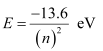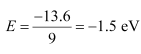For n = 3,
This energy is approximately equal to the energy of gaseous hydrogen. It can be concluded that the electron has jumped from n = 1 to n = 3 level.
During its de-excitation, the electrons can jump from n = 3 to n = 1 directly, which forms a line of the Lyman series of the hydrogen spectrum.
We have the relation for wave number for Lyman series as: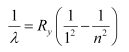Where,
Ry = Rydberg constant = 1.097 × 107 m−1
λ= Wavelength of radiation emitted by the transition of the electron
For n = 3, we can obtain λ as: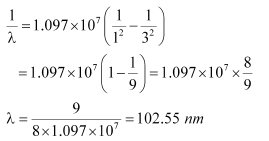If the electron jumps from n = 2 to n = 1, then the wavelength of the radiation is given as: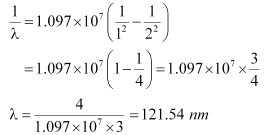If the transition takes place from n = 3 to n = 2, then the wavelength of the radiation is given as: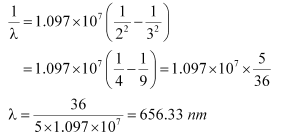This radiation corresponds to the Balmer series of the hydrogen spectrum.

Hence, in Lyman series, two wavelengths i.e., 102.5 nm and 121.5 nm are emitted. And in the Balmer series, one wavelength i.e., 656.33 nm is emitted.

Ques 12.10:

In accordance with the Bohr’s model, find the quantum number that characterises the earth’s revolution around the sun in an orbit of radius 1.5 × 1011 m with orbital speed 3 × 104m/s. (Mass of earth = 6.0 × 1024 kg.)

Ans: Radius of the orbit of the Earth around the Sun, r = 1.5 × 1011 m

Orbital speed of the Earth, ν = 3 × 104 m/s

Mass of the Earth, m = 6.0 × 1024 kg

According to Bohr’s model, angular momentum is quantized and given as: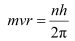Where,

h = Planck’s constant = 6.62 × 10−34 Js

n = Quantum number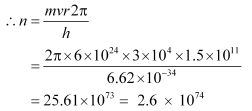Hence, the quanta number that characterizes the Earth’ revolution is 2.6 × 1074.

Ques 12.11:

Answer the following questions, which help you understand the difference between Thomson’s model and Rutherford’s model better.

(a) Is the average angle of deflection of α­-particles by a thin gold foil predicted by Thomson’s model much less, about the same, or much greater than that predicted by Rutherford’s model?

(b) Is the probability of backward scattering (i.e., scattering of α-particles at angles greater than 90°) predicted by Thomson’s model much less, about the same, or much greater than that predicted by Rutherford’s model?

(c) Keeping other factors fixed, it is found experimentally that for small thickness t, the number of α-particles scattered at moderate angles is proportional to t. What clue does this linear dependence on t provide?

(d) In which model is it completely wrong to ignore multiple scattering for the calculation of average angle of scattering of α-particles by a thin foil?

The average angle of deflection of α­-particles by a thin gold foil predicted by Thomson’s model is about the same size as predicted by Rutherford’s model. This is because the average angle was taken in both models.

(b) much less

The probability of scattering of α-particles at angles greater than 90° predicted by Thomson’s model is much less than that predicted by Rutherford’s model.

(c) Scattering is mainly due to single collisions. The chances of a single collision increases linearly with the number of target atoms. Since the number of target atoms increase with an increase in thickness, the collision probability depends linearly on the thickness of the target.

(d) Thomson’s model

It is wrong to ignore multiple scattering in Thomson’s model for the calculation of average angle of scattering of α­−particles by a thin foil. This is because a single collision causes very little deflection in this model. Hence, the observed average scattering angle can be explained only by considering multiple scattering.

Ques 12.12:

The gravitational attraction between electron and proton in a hydrogen atom is weaker than the coulomb attraction by a factor of about 10−40. An alternative way of looking at this fact is to estimate the radius of the first Bohr orbit of a hydrogen atom if the electron and proton were bound by gravitational attraction. You will find the answer interesting.

Ans: Radius of the first Bohr orbit is given by the relation,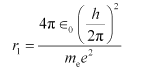Where,

0 = Permittivity of free space

h = Planck’s constant = 6.63 × 10−34 Js

me = Mass of an electron = 9.1 × 10−31 kg

e = Charge of an electron = 1.9 × 10−19 C

mp = Mass of a proton = 1.67 × 10−27 kg

r = Distance between the electron and the proton

Coulomb attraction between an electron and a proton is given as: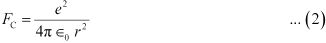Gravitational force of attraction between an electron and a proton is given as: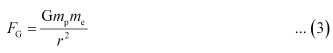Where,

G = Gravitational constant = 6.67 × 10−11 N m2/kg2

If the electrostatic (Coulomb) force and the gravitational force between an electron and a proton are equal, then we can write:

FGFC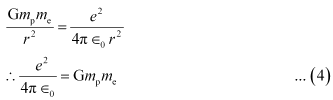Putting the value of equation (4) in equation (1), we get: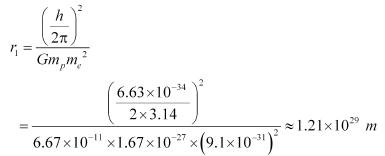It is known that the universe is 156 billion light years wide or 1.5 × 1027 m wide. Hence, we can conclude that the radius of the first Bohr orbit is much greater than the estimated size of the whole universe.

Ques 12.13:

Obtain an expression for the frequency of radiation emitted when a hydrogen atom de-excites from level n to level (n−1). For large n, show that this frequency equals the classical frequency of revolution of the electron in the orbit.

Ans: It is given that a hydrogen atom de-excites from an upper level (n) to a lower level (n−1).

We have the relation for energy (E1) of radiation at level n as: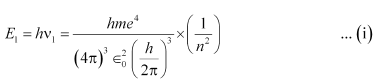Where,
v1 = Frequency of radiation at level n
h = Planck's constant
m = Mass of hydrogen atom
e = Charge on an electron
= Permittivity of free space

Now, the relation for energy (E2) of radiation at level (n − 1) is given as: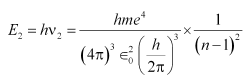......(ii)

Where,
v2 = Frequency of radiation at level (n-1)

Energy (E) released as a result of de-excitation:

EE2E1

E2 − E1 … (iii)

Where,

ν = Frequency of radiation emitted

Putting values from equations (i) and (ii) in equation (iii), we get: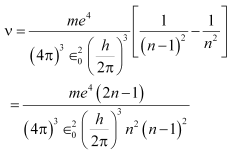For large n, we can write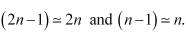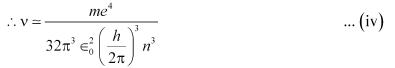Classical relation of frequency of revolution of an electron is given as: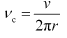Where,

Velocity of the electron in the nth orbit is given as: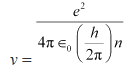....(i)

And, radius of the nth orbit is given as:....(ii)

Putting the values of equations (vi) and (vii) in equation (v), we get: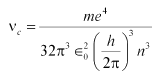....(iii)

Hence, the frequency of radiation emitted by the hydrogen atom is equal to its classical orbital frequency.

Ques 12.14:

Classically, an electron can be in any orbit around the nucleus of an atom. Then what determines the typical atomic size? Why is an atom not, say, thousand times bigger than its typical size? The question had greatly puzzled Bohr before he arrived at his famous model of the atom that you have learnt in the text. To simulate what he might well have done before his discovery, let us play as follows with the basic constants of nature and see if we can get a quantity with the dimensions of length that is roughly equal to the known size of an atom (~ 10−10 m).

(a) Construct a quantity with the dimensions of length from the fundamental constants eme, and c. Determine its numerical value.

(b) You will find that the length obtained in (a) is many orders of magnitude smaller than the atomic dimensions. Further, it involves c. But energies of atoms are mostly in non-relativistic domain where c is not expected to play any role. This is what may have suggested Bohr to discard c and look for ‘something else’ to get the right atomic size. Now, the Planck’s constant h had already made its appearance elsewhere. Bohr’s great insight lay in recognising that hme, and e will yield the right atomic size. Construct a quantity with the dimension of length from hme, and e and confirm that its numerical value has indeed the correct order of magnitude.

Ans: (a) Charge on an electron, e = 1.6 × 10−19 C

Mass of an electron, me = 9.1 × 10−31 kg

Speed of light, c = 3 ×108 m/s

Let us take a quantity involving the given quantities as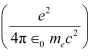Where,

0 = Permittivity of free space

And,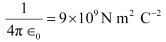The numerical value of the taken quantity will be: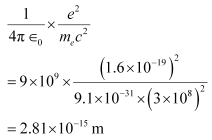Hence, the numerical value of the taken quantity is much smaller than the typical size of an atom.

(b) Charge on an electron, e = 1.6 × 10−19 C

Mass of an electron, me = 9.1 × 10−31 kg

Planck’s constant, h = 6.63 ×10−34 Js

Let us take a quantity involving the given quantities as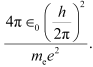Where,

0 = Permittivity of free space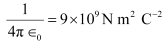And,

The numerical value of the taken quantity will be: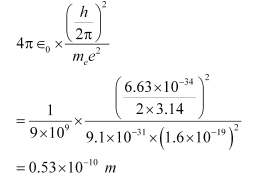Hence, the value of the quantity taken is of the order of the atomic size.

Ques 12.15:

The total energy of an electron in the first excited state of the hydrogen atom is about −3.4 eV.

(a) What is the kinetic energy of the electron in this state?

(b) What is the potential energy of the electron in this state?

(c) Which of the answers above would change if the choice of the zero of potential energy is changed?

Ans: (a) Total energy of the electron, E = −3.4 eV

Kinetic energy of the electron is equal to the negative of the total energy.

K = −E

= − (− 3.4) = 3.4 eV

Hence, the kinetic energy of the electron in the given state is 3.4 eV.

(b) Potential energy (U) of the electron is equal to the negative of twice of its kinetic energy.

U = −2 K

= − 2 × 3.4 = − 6.8 eV

Hence, the potential energy of the electron in the given state is − 6.8 eV.

(c) The potential energy of a system depends on the reference point taken. Here, the potential energy of the reference point is taken as zero. If the reference point is changed, then the value of the potential energy of the system also changes. Since total energy is the sum of kinetic and potential energies, total energy of the system will also change.

Ques 12.16:

If Bohr’s quantization postulate (angular momentum = nh/2π) is a basic law of nature, it should be equally valid for the case of planetary motion also. Why then do we never speak of quantization of orbits of planets around the sun?

Ans: We never speak of quantization of orbits of planets around the Sun because the angular momentum associated with planetary motion is largely relative to the value of Planck’s constant (h). The angular momentum of the Earth in its orbit is of the order of 1070h. This leads to a very high value of quantum levels n of the order of 1070. For large values of n, successive energies and angular momenta are relatively very small. Hence, the quantum levels for planetary motion are considered continuous.

Ques 12.17:

Obtain the first Bohr’s radius and the ground state energy of a muonic hydrogen atom [i.e., an atom in which a negatively charged muon (μ) of mass about 207me orbits around a proton].

Ans: Mass of a negatively charged muon,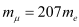According to Bohr’s model,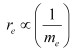And, energy of a ground state electronic hydrogen atom,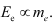We have the value of the first Bohr orbit,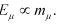Let rμ be the radius of muonic hydrogen atom.

At equilibrium, we can write the relation as: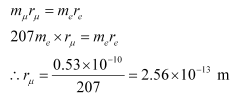Hence, the value of the first Bohr radius of a muonic hydrogen atom is

2.56 × 10−13 m.

We have,

Ee= − 13.6 eV

Take the ratio of these energies as: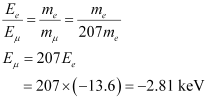Hence, the ground state energy of a muonic hydrogen atom is −2.81 keV.

Offer running on EduRev: Apply code STAYHOME200 to get INR 200 off on our premium plan EduRev Infinity!

## Physics For JEE

188 videos|517 docs|265 tests

,

,

,

,

,

,

,

,

,

,

,

,

,

,

,

,

,

,

,

,

,

;